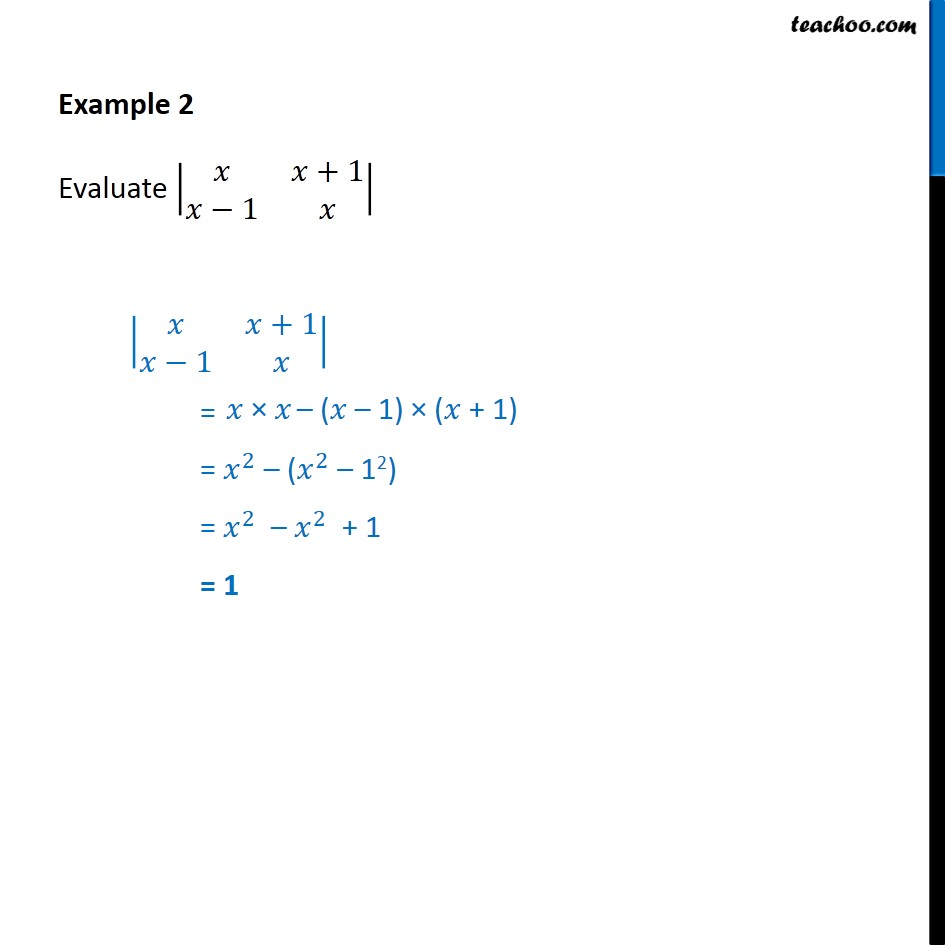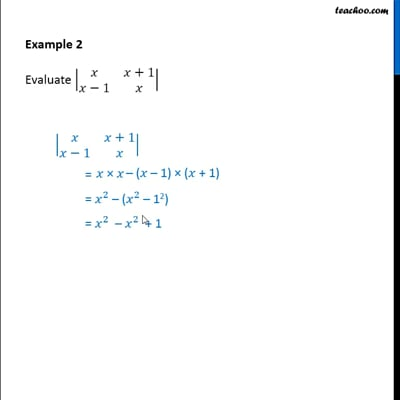Examples

Chapter 4 Class 12 Determinants
Serial order wiseThis video is only available for Teachoo black users

Get live Maths 1-on-1 Classs - Class 6 to 12

### Transcript

Example 2 - Chapter 4 Class 12 Determinants - NCERT Book Evaluate |x x+1 x-1 x| |x x + 1 x - 1 x| = x(x) - (x-1) (x+1) = x^2 – (x^2 - 1^2) = x^2 - x^2 + 1 = 1